# Sample Data: Gene Sequences

Splice-junction Gene Sequences for Primate DNA

Splice junctions are points on a DNA sequence at which "superfluous" DNA is removed during the process of protein creation in higher organisms. The problem posed in this dataset is to recognize, given a sequence of DNA, the boundaries between exons (the parts of the DNA sequence retained after splicing) and introns (the parts of the DNA sequence that are spliced out). In the biological community, intron/exon borders are referred to a "acceptors" while exon/intron borders are referred to as "donors".

## Examples

### Basic Examples

Retrieve the resource:

 In:=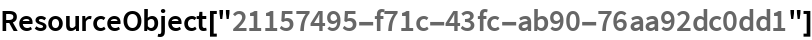Out=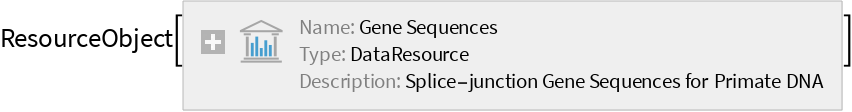Retrieve the default content:

 In:=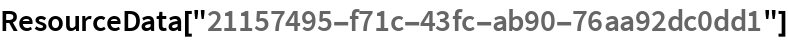Out=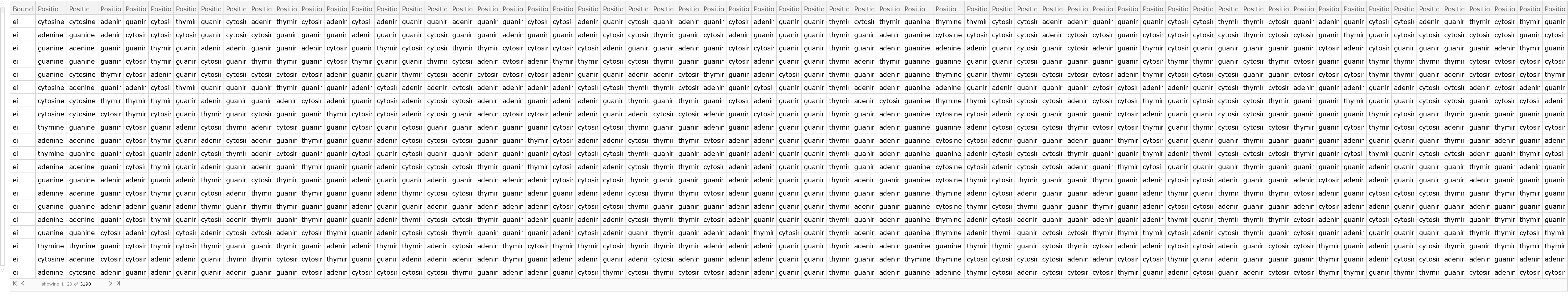### Analysis

Shuffle the dataset randomly:

 In:=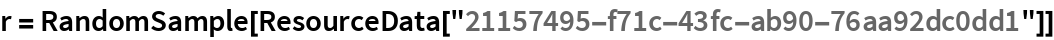Out=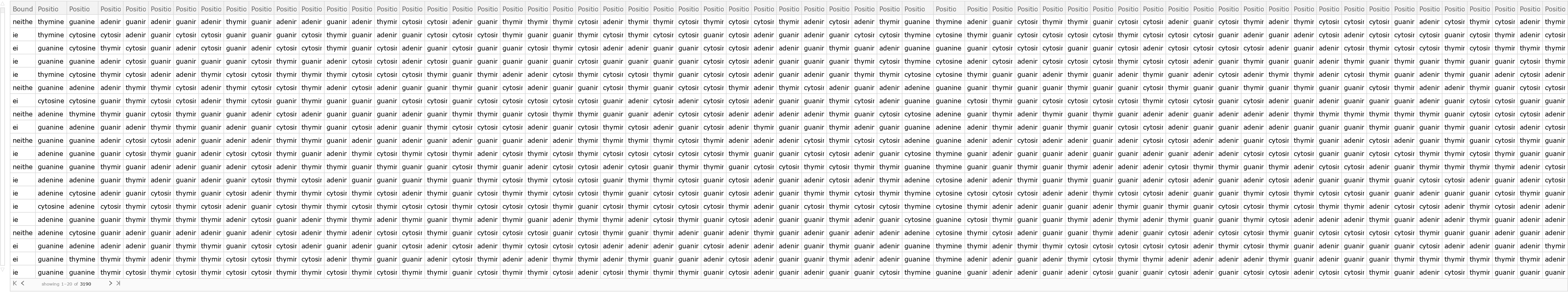Create a training dataset using 80% of the original dataset:

 In:=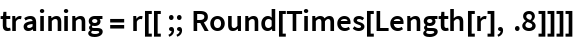Out=Create a testing dataset using the remaining 20% of the original dataset:

 In:=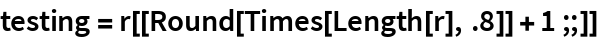Out=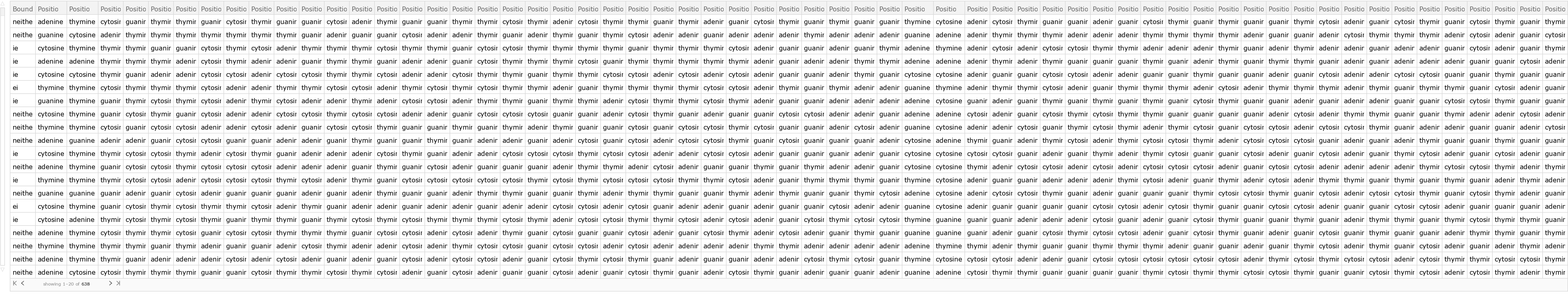Train a classifier:

 In:=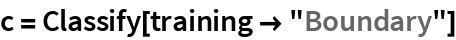Out=Obtain general information about the classifier:

 In:=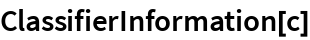Out=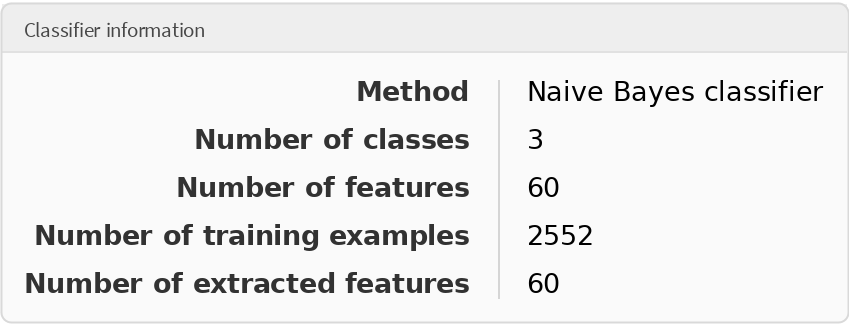Generate a ClassifierMeasurementsObject of the classifier with the test set:

 In:=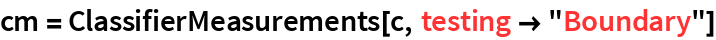Out=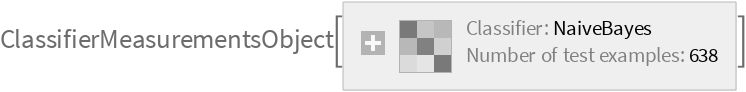Visualize the accuracy of the classifier:

 In:=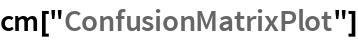Out=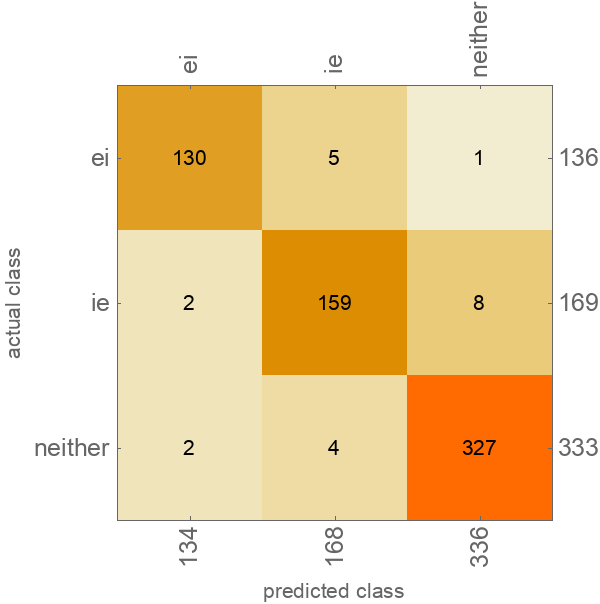Wolfram Research, "Sample Data: Gene Sequences" from the Wolfram Data Repository (2018)